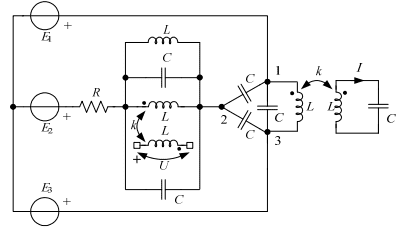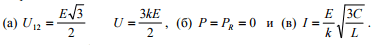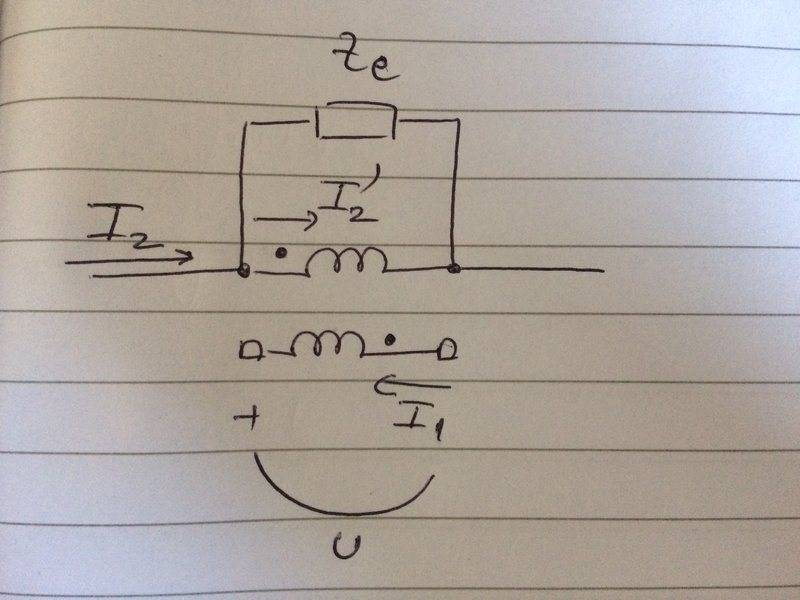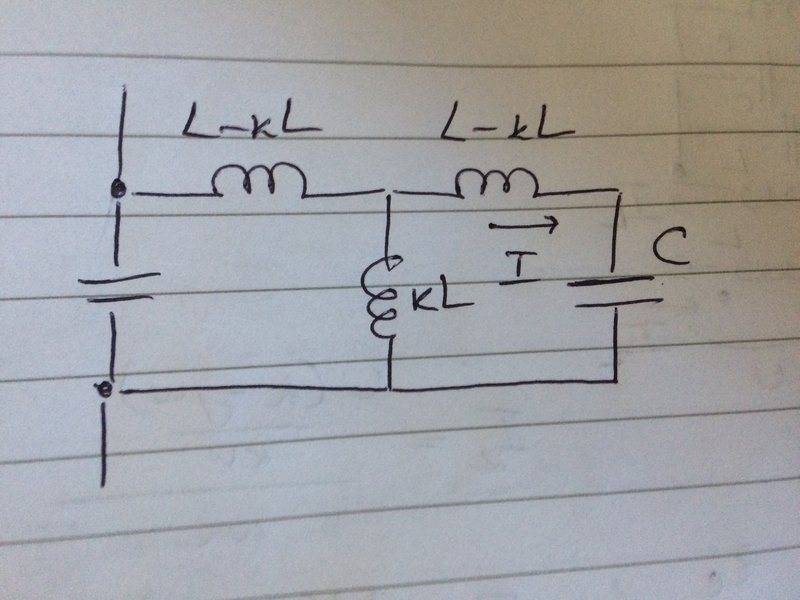# Calculate the effective values in this 3-Phase Circuit

• Engineering
diredragon

## Homework StatementGiven the known quantities ##R, C, L##, ##k##, and the effective values of the voltages ##E_1=E_2=E_3=E## , ##w = \frac{1}{\sqrt{LC}}## of the direct-symmetrical 3-Phase system find the expressions for the effective values of ##U_{12}##, ##U## and ##I## and calculate the active power ##P## of the 3-Phase generator.

## Homework Equations

3. The Attempt at a Solution [/B]
Let me write down the solutions to this problem so we know what to aim for when solving it:I feel kinda overwhelmed to not even know where to start from. I think can express the current ##I_2## of the generator ##E_2## in terms of the total impedance of the middle part and the voltage ##U##.##U=jwI_1L+jwkLI'_2 => I'_2 = \frac{U}{jwkL}## since ##I_1=0##. The ##Z_e## in the diagram equals ##Z_e = jwL||\frac{1}{2jwC} = -jwL##.
The current ##I'_2 = I_2\frac{Z_e}{Z_e+L} => I_2 = \frac{U(k-jw)}{kLw^2}## and this is all i can get out of this first part. I could also make the left part like this:But i don't see how that would help. What am i missing? Is there a course of action to take that i don’t see here?

#### Attachments

Homework Helper
Gold Member
First look at the middle LC network. One of the coupled inductors is open-circuited, so it won't have any effect on the main circuit. That leaves you with 2 Ls and 2 Cs, all in parallel. What would be their effective reactance?

diredragon
First look at the middle LC network. One of the coupled inductors is open-circuited, so it won't have any effect on the main circuit. That leaves you with 2 Ls and 2 Cs, all in parallel. What would be their effective reactance?
I get ##\frac{jwL}{2}||\frac{1}{2jwC} = \frac{-jwL}{2(1-wL)} = \frac{-jL}{2(\sqrt{LC}-L)}##

Homework Helper
Gold Member
No.

What can you say about Xc and XL given that ω=1/√LC? What would be the equivalent reactance of the LC network?

•diredragon
diredragon
No.

What can you say about Xc and XL given that ω=1/√LC? What would be the equivalent reactance of the LC network?
Oh, ##X_L = X_C##. So at the points where ##Z_e## should be is open circuit, no current ##I_2## in that branch and since the only resistive element is in that branch that explains ##P = P_R = 0##. The voltage on the two capacitors must be the same as the same current goes through them and that's how i got ##U_{12}##. One thing about ##I##, is there an easier way than to turn this into a 3 inductors on the right side as drawn on the picture in the original post?